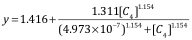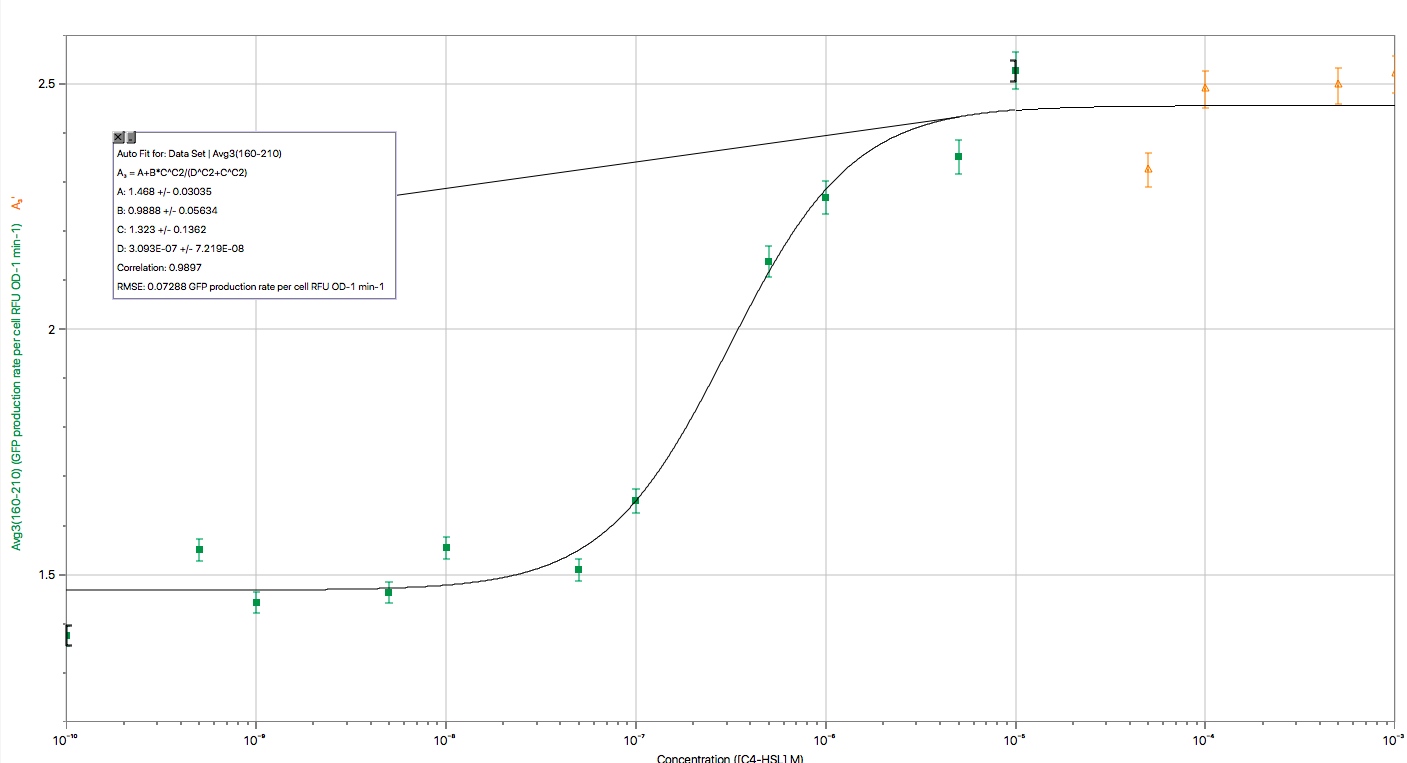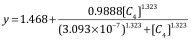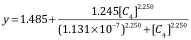# Model

## I. Summary

Our model helped us to optimize the A. hydrophila sensor devices. At first, we measured the concentration of C4-HSL in A. hydrophila culture by using mass spectrum. Then, we tested the GFP production rate of sensor device I. The experimental results were characterized by using Hill equation, which modelled the GFP synthesis rate as a function of input concentration of the inducer, C4-HSL. However, when we predicted the efficiency of this device in real environment by the derived function, we found the fluorescence was too low to be detected. Therefore, we adjusted our design by increasing rhlR RBS strength and modelled the experimental results by using Hill equation again. This time, we found the device’s (BBa_K2548001) fluorescence in real environment was enough to be detected. More importantly, our model can help to indicate the A. hydrophila concentration in different environments, and alerts the aquaculture managers the danger of pathogen infection. In addition, we visualize the data in three-dimensions to show how GFP production rate per cell over time at different C4-HSL inducer concentrations to characterize the sensor in a more comprehensive way.

## II. Assumptions

1. When the concentration of   changes, the synthesis rate of GFP increases, and its fluorescence increases.
2. GFP synthesis rate is only affected by the concentration of C4-HSL, and the relationship can be simplified into a non-linear function (Hill equation).
3. The function of relevant proteins is assumed stable throughout the experiment.
4. The difference between individual subtype of bacteria is omitted.

## III. Design of Characterizations

In order to characterize the expression system, we first acquired the experiment data of GFP production rate per cell (RFU per Abs per min) under different concentration of inducers(M) (see experiment). Then the experimental data were fitted using the Hill equation:In biochemistry, the binding ability of a ligand to a macromolecule is often increased if other ligands have already present on the same macromolecule. The hill equation is used to determine the cooperativeness of a ligand binding to its receptor, and it can describe the relationship between the expression level of genes which are regulated and the quantity of regulatory factors.

We model the GFP synthesis rate () as a function of input concentration of(). The four parameters (,,,) were estimated to obtain the best fit curve by performing a non-linear curve fitting using the experimental data. Among the parameters, n is the Hill coefficient, which describes the cooperativity; C is the concentration of C4-HSL (M) which produces half occupation. This curve fitting was performed using Logger Pro. (Saeidi et al, 2011).

## IV. Results of Characterizations of Sensor Device I and Device IIFig 1. Characterization of Sensor Device I (BBa_K2548000 + BBa_K2548003)

The figure above shows the modeling result of Device I. The derived equation is:And the correlation coefficient of the equation is 0.9816.Fig 2. Characterization of Sensor Device II

The figure above shows the modelling result of Device II. The derived equation is:And the correlation coefficient of the equation is 0.9897.Fig 3. Characterization of Sensor Device II (BBa_K2548001 + BBa_K2548003)

The figure above shows the modeling result of Device III. The derived equation is:And the correlation coefficient of the equation is 0.9952.

We used the two equations to predict the efficiency of the sensor devices. See the analysis of these results at demonstrate.

## V. Visualization of Data in Three-Dimensions

The GFP production rate is converted as RFU per Abs per min, calculated through the arithmetic average of three trails to eliminate the systematic and experimental errors. The three axes are named respectively as Time (min),, and GFP production rate. The three variables are defined respectively in different sets, automatically imported from Excel to maintain the efficiency and productivity of modeling.

Time( t ) ∈ [ 0,220 ] with interval of 10mins .

C4-HSL concentration ( M ) ∈ [,] for geometric sequence of ratio of 5 . To maintain the integrity of image, the value of concentration is replaced by marks from 1-15.

GFP production rate ∈ [ 0,4 ] from experimental test experience.

The modeling is achieved through Mathematica 11. All discrete points are contained in manual data set. To show the variation tendency of data, we took MeshFunction to connect the adjacent points and present the regional changing trend, like the concavity or convexity. To specify and highlight the progressive changes of GFP production rate, we took the PlotLegends Function of Colorful pattern. The three-dimension graph can be rotated 360 degrees and observed from different views.Fig 4. Visualization of Sensor Device I Experiment DataFig 5. Visualization of Sensor Device III Experiment Data

To illustrate the three-dimensional data in plane and the height ( z -axis value) is represented through the shade of color. The image is generated through ListDensityPlot Function.Fig 6. Visualization of Sensor Device I Experiment Data in PlaneFig 7. Visualization of Sensor Device III Experiment Data in Plane

# References

Saeidi, N., Wong, C. K., Lo, T., Nguyen, H. X., Ling, H., Leong, S. S., . . . Chang, M. W. (2014).        Engineering microbes to sense and eradicate Pseudomonas aeruginosa, a human pathogen. Molecular Systems Biology, 7(1), 521-521. doi:10.1038/msb.2011.55